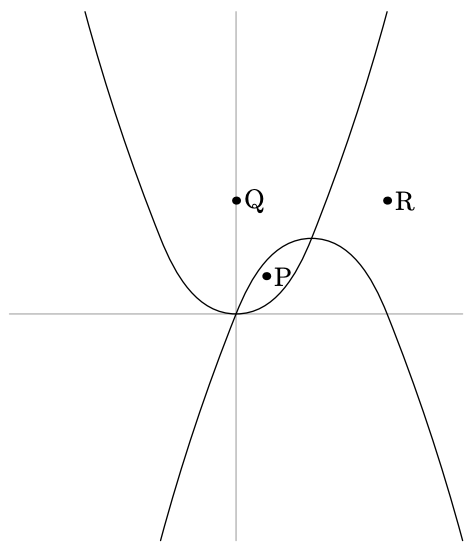시간 제한메모리 제한제출정답맞힌 사람정답 비율
1 초 512 MB20000.000%

## 문제

On a plane there is a set of parabolas given by equations in the form $y = a\cdot x^2 + b\cdot x + c$.

Let's consider a point to be located inside a parabola if it located above the parabola in case of positive coefficient $a$, or below the parabola in case of negative $a$.On this figure, the point $P$ is located inside both parabolas, the point $Q$ is inside one of them, and the point $R$ is inside none of them.

You need to find any point that is located inside all parabolas. It is guaranteed that such point exists.

## 입력

The first line contains a single integer $n$ ($1 \leq n \leq 100\,000$) --- the number of parabolas.

Each of the next $n$ lines contains three integers $a$, $b$, $c$ ($|a|, |b|, |c| \leq 10^9$; $a \neq 0$), describing a parabola $y = a\cdot x^2 + b\cdot x + c$.

## 출력

Print two real numbers $x$ and $y$ --- coordinates of a point located inside all parabolas.

The answer is considered correct if there exists a point at distance at most $10^{-6}$ from the printed one, which is located strictly inside all parabolas.

## 예제 입력 1

4
1 2 3
1 -3 -5
-1 3 4
-2 4 6


## 예제 출력 1

0.24999999632501932 4.124999990812548